# tkinter多选按钮checkbutton讲解

### Checkbutton创建及判断选中状态

```from tkinter import *

def selectjob(): # 判断函数
str='我的选择：'  # 设选择文本的初值
if var1.get()==1:  # 获取变量1的值，为1即勾选了，为0就是没有勾选
str=str+' '+ch1['text']
if var2.get()==1:  # 获取变量2的值，为1即勾选了，为0就是没有勾选
str=str+' '+ch2['text']
print(str)  # 输出选择文本

root=Tk()

var1=IntVar() # 创建整型变量1
var2=IntVar(value=1) # 创建整型变量2，设初值为1，即勾选

ch1=Checkbutton(root,text="足球",variable=var1) # ch1绑定var1
ch1.pack()
ch2=Checkbutton(root,text="排球",variable=var2) # ch1绑定var2
ch2.pack()

but1=Button(root,text="判断",command=selectjob) # 绑定判断函数
but1.pack()

root.mainloop()```

跟上篇文章介绍的单选按钮，区别在于，有几个多选按钮，就先创建几个不同的整形型变量（或者布尔型变量），然后在创建多选按钮时，分别绑定不同的变量。在判断时，只要用变量的get()方法得到的值为1（或者为True），就说明这个多选按钮被选择了。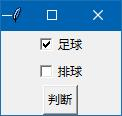```from tkinter import *

def selectjob(): # 判断函数
str='我的选择：'  # 设选择文本的初值
if var1.get()==True:  # 获取变量1的值，为True即勾选了
str=str+' '+ch1['text']
if var2.get()==True:  # 获取变量2的值，为True即勾选了
str=str+' '+ch2['text']
print(str)  # 输出选择文本

root=Tk()

var1=DoubleVar() # 创建布尔型变量1
var2=DoubleVar(value=True) # 创建布尔型变量2，设初值为True，即勾选

ch1=Checkbutton(root,text="足球",variable=var1) # ch1绑定var1
ch1.pack()
ch2=Checkbutton(root,text="排球",variable=var2) # ch1绑定var2
ch2.pack()

but1=Button(root,text="判断",command=selectjob) # 绑定判断函数
but1.pack()

root.mainloop()```

```from tkinter import *

def selectjob(): # 判断函数
str='我的选择：'  # 设选择文本的初值
if var1.get()==True:  # 获取变量1的值，为1即勾选了，为0就是没有勾选
str=str+' '+ch1['text']
if var2.get()==True:  # 获取变量2的值，为1即勾选了，为0就是没有勾选
str=str+' '+ch2['text']
print(str)  # 输出选择文本

root=Tk()

var1=DoubleVar() # 创建布尔型变量1
var2=DoubleVar(value=True) # 创建整型变量2，设初值为1，即勾选

ch1=Checkbutton(root,text="足球",variable=var1,command=selectjob)
ch1.pack()
ch2=Checkbutton(root,text="排球",variable=var2,command=selectjob)
ch2.pack()

root.mainloop()```

运行后，点击任何一个多选按钮，都会触发selectjob函数，得到多选按钮的勾选情况。

### 改变Checkbutton多选按钮样式

跟单选按钮一样，只要在创建多选按钮时，增加参数 indicatoron=0 就可以改变按钮的外观，让多选按钮像普通按钮一样。

上面的代码改一下。源码及文章来自 wb98.com 济亨网

```from tkinter import *

root=Tk()

var1=DoubleVar() # 创建布尔型变量1
var2=DoubleVar(value=True) # 创建整型变量2，设初值为1，即勾选

ch1=Checkbutton(root,text="足球",variable=var1,indicatoron=0) # ch1绑定var1
ch1.pack()
ch2=Checkbutton(root,text="排球",variable=var2,indicatoron=0) # ch1绑定var2
ch2.pack()

root.mainloop()```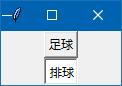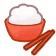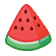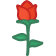```from tkinter import *

root=Tk()

var1=DoubleVar() # 创建布尔型变量1
var2=DoubleVar(value=True) # 创建整型变量2，设初值为1，即勾选
var3=DoubleVar()

img1=PhotoImage(file='dfg.png') # 创建图像对象
img2=PhotoImage(file='dfi.png')
img3=PhotoImage(file='ebn.png')

ch1=Checkbutton(root,text="米饭",image=img1,variable=var1) # ch1绑定var1
ch1.pack()
ch2=Checkbutton(root,text="西瓜",image=img2,variable=var2) # ch1绑定var2
ch2.pack()
ch3=Checkbutton(root,text="鲜花",image=img3,variable=var3) # ch1绑定var2
ch3.pack()

root.mainloop()```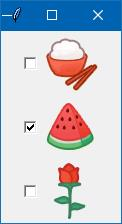compound有多个选项：left, right, top, bottom, center

```from tkinter import *

root=Tk()

var1=DoubleVar() # 创建布尔型变量1
var2=DoubleVar(value=True) # 创建整型变量2，设初值为1，即勾选
var3=DoubleVar()

img1=PhotoImage(file='dfg.png') # 创建图像对象
img2=PhotoImage(file='dfi.png')
img3=PhotoImage(file='ebn.png')

ch1=Checkbutton(root,text="米饭",image=img1,compound='left',variable=var1) # 图像显示在文本的左边
ch1.pack()
ch2=Checkbutton(root,text="西瓜",image=img2,compound='right',variable=var2) # 图像显示在文本的右边
ch2.pack()
ch3=Checkbutton(root,text="鲜花",image=img3,compound='left',variable=var3) # 图像显示在文本的左边
ch3.pack()

root.mainloop()```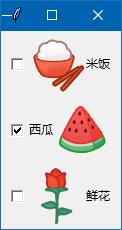```from tkinter import *

root=Tk()

var1=DoubleVar() # 创建布尔型变量1
var2=DoubleVar(value=True) # 创建整型变量2，设初值为1，即勾选
var3=DoubleVar()

img1=PhotoImage(file='dfg.png') # 创建图像对象
img2=PhotoImage(file='dfi.png')
img3=PhotoImage(file='ebn.png')

ch1=Checkbutton(root,text="米饭",image=img1,compound='left',variable=var1,relief='groove') # 图像显示在文本的左边
ch1.pack()
ch2=Checkbutton(root,text="西瓜",image=img2,compound='right',variable=var2,relief='groove') # 图像显示在文本的右边
ch2.pack()
ch3=Checkbutton(root,text="鲜花",image=img3,compound='left',variable=var3,relief='groove') # 图像显示在文本的左边
ch3.pack()

root.mainloop()```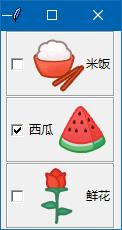<< 上一篇 下一篇 >>